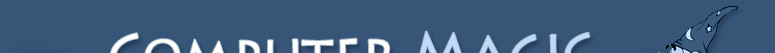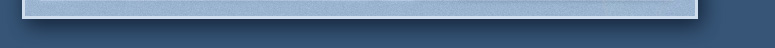{ }

## PHP Tutorial – Lesson 7 You must choose!

When learning to write software, one of the most fundamental things is to make decisions. Usefull software requires input. Input is simply a clue to the program as to what to do next. For instance, when you are playing your favorite FPS (First Person Shooter), and you are running around shooting up all the rabid sheep and leaving bloody wool all over the place, the input you give to the game is via the keyboard and mouse. When you push the forward key, your guy moves forward, when you push the back key, your guy moves back. Input. Output in this case would be the visual representation of the world, which changes based on your movement (input). Input and Output comes in many forms (command line programs, point and click GUI, 3D games, etc…) but it is all conceptually the same, the input informs the application what the user intends.

Once you have that input, you need to decide what to do with it. Now I know the user pushed the OK button, or the user pushed the up arrow, what should I do now? In the case of an FPS game, you might want to only move forward if they player is alive and kicking. This would require a choice, is the players health above 0? If it is, then we move forward, if not, we are a bloody mess on the pavement, so make like a dead guy and stay. Here is some pseudo code (pseudo code is code that captures the logic, but isn’t really a specific language and wouldn’t actually work).

``````
if (player_health > 0) then
Move(FORWARD)
else
return 'Do nothing!'
end if
```
```

Ok, now, this pseudo code looks more like Basic than PHP! Why would I do that in a PHP tutorial? For those seasoned programmers out there, this is dumb, but for newbies, this is more readable. Don’t worry, I will show the PHP version in a minute.

Also worth noting is the concept of code blocks. A chunk of code that logically goes together is called a block. In the previous example, the blocks were one line each (e.g. Move(FORWARD)). Using the concept of blocks, you can have one or 100 lines in a code block, it doesn’t matter. The kewords THEN, ELSE, and END IF signal that a block is starting (THEN), another new block is starting and the previous block is ending (ELSE), and that the last block is ending (END IF).

Lets break this down now. We make choices by using the IF statement. The IF statement lets us tell the computer to execute a certain block of code only if the expression is true. This is important. The IF statement doesn’t care what you are comparing or what is happening inside the expression, it only cares if the end result is True or not.

``````
if (expression) then...
DO THIS
end if
```
```

The portion of this code “DO THIS” will ONLY be executed if the expression is True. Is 5 = to 5? It sure is, so if the expression was…

``````
if (5 == 5) then
THIS WOULD EXECUTE
end if
```
```

Notice that a double equals was used for comparison. Most languages do this so that the language can tell if you are trying to assign the value of 5 to five (5=5) or trying to compare the two (5 == 5). In the expression, you can put any valid components as long as they result in a true or false answer (>, < , <=, >=, ==, !=, etc..). The “DO THIS” section of the code ONLY executes if the expression evaluates to true. Since 5 always equals 5, then that expression is always true. If you were to try (6 == 5), then the expression would ALWAYS be false and the “DO THIS” section of code would be skipped. The trailing END IF key words tell where the True block of code ends so the language knows how far down to skip if the expression is False.

Some times you want to know when the expression is false. To do this, you can use the NOT key word (the ! symbol in PHP represents the NOT keyword). Here is an example.

``````
if (6 != 5) then
THIS WILL EXECUTE!
end if
```
```

By throwing in the != instead of the ==, we are flipping the test. The “DO THIS” section still only executes if the expression is true. Normally, 6 == 5 would be false, but when you put the NOT symbol in there, you are saying “does 6 not equal 5?”. This statement is true. Read that a few times to get your head wrapped around it.

Ok, now for another example. What if you want to take specific action based on a true result, and a different action based on a false result (like with our first example). You can use the ELSE keyword to create a false code block.

``````
if (5 == 5) then
TRUE BLOCK
else
FALSE BLOCK
end if
```
```

The first block is ALWAYS the True block (even if you use the NOT symbol in your expression, the True block only executes if the expression is true). The block after the ELSE keyword is a catch all. It basically picks up what is left over. If the True block executes, then it will skip over the False block. If the True block does not execute, it will execute the False block. The else (False block) is optional as you may have noticed in some of the previous examples.

Now that we have looked at some examples of pseudo code, lets look at an example of PHP code that really works.

``````
<?php
\$name = \$_GET["name"];
\$age = \$_GET["age"];

if (\$age < 12) {
print "\$name whats up kid?";
} elseif (\$age > 11 && \$age < 30) {
print "\$name dude, would you like some fries with that?";
} else {
print "\$name sir, yes sir!";
}
?>
```
```

Notice that in PHP you replace the If/THEN with an IF {. The { symbol indicates the start of a block of code. The } symbol indicates the end of a block of code. The else keyword is still used, but notice that it is preceded by a } to close the previous block of code, and followed by a { to indicate the starting of a new block of code. All programming languages are the same in the way the IF statements work, the only real difference is what symbol or keyword they use to indicate the start and end of a block of code.

The tricky part here is that the ELSEIF keyword is used in the second expression. This allows me to have two or more True blocks, each with different conditions. One thing to note here is that once any one of the blocks (True or False) is executed, execution jumps down to the END IF statement (or final } symbol). This means that if the first expression is True and that block executes, the second block will NEVER execute even if the second expression is true also. In this can, only one of the three blocks will ever execute.

And lastly, I got even more tricky by putting more than one expression after the ELSEIF keyword. This allows me to check more than one thing at a time. In this case, I could check to make sure the age specified was in a particular range by using the && symbol (which stands for AND). By using the AND method to combine two expressions, this means that BOTH expressions have to be true for the final response to be True. Should either expression be False, the entire final expression will result in a False answer. If you wanted to execute the current block if either expression were true, you could use the symbol for OR which is ||. This would mean that if the first expression is true, OR the second expression is true, then the result is True. This can complicate things greatly and further discussion will be saved for a future article. Feel free to tare out your hair trying to figure it out on your own though 🙂

To see how this script works, try uploading it to your server and requesting the file. If you put nothing on the address line (except the URL to the script) you will get something like this (you will probly also get a couple warnings):

``````
sir, yes sir!
```
```

Since you didn’t specify an age, it use the catch all (ELSE clause) and will print the message without your name. Try adding this to the end of the URL.

``````
?name=bob&age=5
```
```

You should now get a different message. Try changing the age around to get different messages and see how the IF statements work.

Ray Pulsipher

Owner

Computer Magic And Software Design